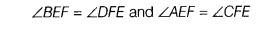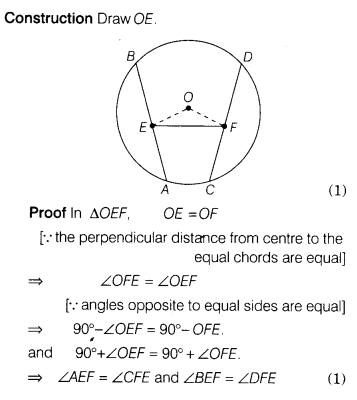# Prove that the line joining the mid-points of two equal chords of circle subtends equal angles

Prove that the line joining the mid-points of two equal chords of circle subtends equal angles with the chord.

Since, AS and CD are two equal chords of a circle with centre O. E and P are the mid-points of AS and CD, respectively.